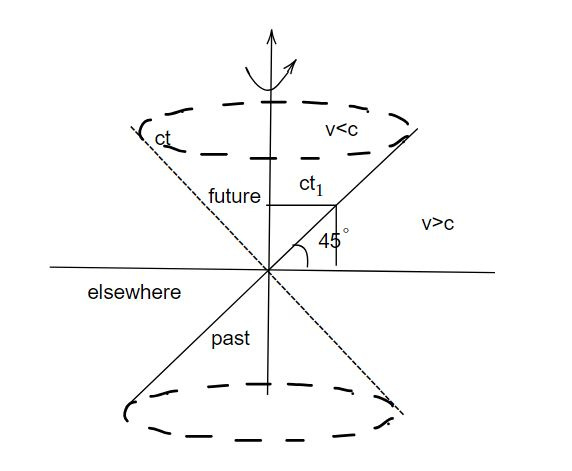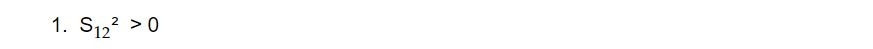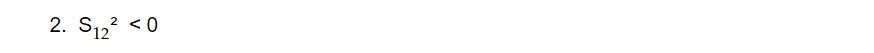# Four dimensional space and light cone

Four-dimensional space, often denoted as 4D space, is a mathematical concept used in various fields such as physics and mathematics. It represents a space with four dimensions, typically labeled as x, y, z, and t. The first three dimensions (x, y, z) are spatial dimensions, which describe the physical position of an object in three-dimensional space. The fourth dimension (t) represents time. Together, these four dimensions create a framework in which events and objects can be located both spatially and temporally.

One important concept related to four-dimensional space in the context of special relativity in physics is the "light cone." The light cone is a geometric construct used to describe how information (including light signals) spreads through spacetime, taking into account the finite speed of light. It is a fundamental concept in understanding causality and the structure of spacetime in the theory of special relativity, as developed by Albert Einstein. In LT we have four coordinates ct, x, y, z. The space formed by these four coordinate is called four dimensional space. To represent it, we use two dimensional space along y axis, we take ct and x along x axis. Other two are supposed ct

There are two displacement

1. Time displacement (ct)

2. Space displacement (x, y, z)

ds² is invariant

Since ds² = c²dt² - dx² -dy²-dz²

speed of light is upper limit of speed i.e

For material particle v<c

In time t, time displacement = ct

space displacement = vt

ct > vt

A point in 4 dimensional represents an event. So a line in this space represents succession of events. The line is called world line. For photon, v=c

time displacement = ct_1

space displacement = ct_2For light, the world is a straight line through origin. If we imagine that the figure is rotated about ct axis.

The world line for the light describes a cone called light cone. The events outside the light cone are not observed so the region outside the light cone is called elsewhere.

The upper part of light cone is future and lower part is past. Let us consider two events P_1(x_1, t_1) and P_2(x_2, t_2) in space time.

Then space time intervalThere are possibilitiesIn this case we can find Lorentz transformation to a new systemSuch events are called time like separation. That is two events separated by time. Two events are said to be time-like separated if the spacetime interval between them is positive and less than the square of the speed of light (c^2). Time-like separated events are causally connected, which means one event can influence the other, and this influence can propagate at or below the speed of light. An observer traveling between these events at sub-light speeds can experience them in a definite time order.

• Examples of time-like separated events include events in the life of an observer or events connected by the motion of a massive object, like a spaceship traveling from one point in spacetime to another.We find find Lorentz transformation to a new system such that t_1 '' = t_2'' thenThe events are said to be space like separation. Space-like separated events are not causally connected in the sense that no information or influence can travel between them at any speed. They are outside each other's light cones and do not have a definite time order in all reference frames.

• Examples of space-like separated events include events at different locations in spacetime that cannot be connected by any causal signal traveling at or below the speed of light.The events lie on the light cone and are said to have light like separation. These events are connected by only light signal. Light-like separated events lie on the boundary between time-like and space-like separations. Light-like intervals represent the path that a photon (a particle of light) would take between the events.

• Light-like separated events are connected by a photon's trajectory and are typically associated with electromagnetic interactions.

In summary, time-like separation indicates causally connected events that can influence each other within the speed-of-light limit, while space-like separation represents events that are not causally connected and have no definite time order in all reference frames. Light-like separation corresponds to events connected by the path of light itself and is right on the boundary between time-like and space-like separations. These distinctions are fundamental to the relativistic description of spacetime in the theory of special relativity.

This note is a part of the Physics Repository.